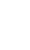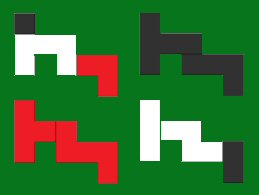Ten-Yen:  the four-quads problemHere is an intriguing question. The total area of Ten-Yen is 36 squares. Can they be divided into four equal groups of 9 and formed into congruent shapes? With tenacious searching, both Kate Jones and Robert Vermillion found the three ways to do this. Out of the 1285 possible shapes of 9 squares, here are the three that work:In October 2014, Robert Vermillion verified that these are indeed the only 3 possible divisions of the Ten-Yen pieces that yield a quartet of identical shapes, most of them consisting of more than one color. Without referring to these solutions, can you build a quartet of congruent shapes on your own?